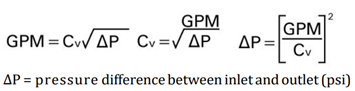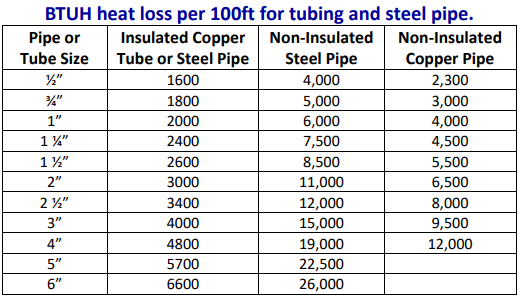# CircuitSolver Design Guide

### Important Note:

To determine pressure loss in the recirculation system, we recommend the use of traditional pipe sizing and head loss practices. Design guides published by ASPE and ASHRAE are widely accepted and recommended. The following guide is not intended as a design recommendation when specifying CircuitSolver valves, only as a guide to allow a plumbing designer to use any values of their choice.

### Calculating Pressure Loss Across the Valve:

To calculate the pressure loss across the CircuitSolver®, use the “Design Cv” shown in the chart below. This Cv is typical for a CircuitSolver® valve under normal working conditions. Use the value that you calculate for the branch design GPM required to offset heat loss in the equation below the chart. Include that pressure drop in your head loss calculations for the circuit and pump sizing.

 CircuitSolver size, NPT Cv fully open Cv “closed” Design Cv ½” 1.3 0.2 0.6 ¾” 1.8 0.2 0.85 1″ 3.3 0.2 1.57 1¼” 5.1 0.2 2.48 1½” 7.6 0.2 3.72 2″ 14.2 0.2 7.02

Flow rate calculation using Cv factor shown.### Recirculation Pump Sizing:

In a recirculation system, the pump must be sized to provide sufficient flow to compensate for the total heat loss in all the supply branches to the furthest fixture in each circuit. Heat loss in the return lines downstream of the CircuitSolvers is not included in the flow rate calculations.

The required flow rate formula is: GPM = BTUh/(ΔT X 500)

A common design practice for domestic hot water recirculation systems is to use ΔT = 5°F. This is the temperature difference of the recirculating water between the heat source and the furthest fixture in each circuit. If we assume this common value of a ΔT = 5°F, the above equation simplifies to GPM = BTUh/2500

If the designer prefers a ΔT = 10°F the above equation simplifies to GPM = BTUh/5000

Note that if a smaller temperature drop is allowed, a higher flow rate is required. The BTUH heat loss will vary based on pipe type, size, length, and insulation. The BTUH heat loss table below is used only to demonstrate example calculations and should not be referenced for real world BTUH heat loss.Example 1:

Calculate the recirculation flow rate required for ΔT = 5°F with 100 feet of ¾” insulated copper pipe, and determine the pressure drop across the CircuitSolver® at that flow rate.

Use the chart above for heat loss of 1800 BTU/h per 100 feet and the equation above for temperature drop ΔT = 5°F. Flow rate = 1800 / 2500 = 0.72 GPM flow required in that circuit.

Using a ¾” CircuitSolver® with Design Cv = 0.85: pressure drop = (0.72 / 0.85) 2 = 0.72 PSI Conclusion: Pressure drop across a ¾” CircuitSolver® with design Cv of 0.85 and branch flow of 0.72 GPM is 0.72 PSI.

Example 2:

Calculate the recirculation flow rate required for ΔT = 5°F for 200 feet of ½” insulated copper pipe, and determine the pressure drop across a ½” CircuitSolver® at that flow rate.

Use the chart above for heat loss of 1600 BTU/h per 100 feet (X2 for 200 ft.) and the equation above for temperature drop of ΔT = 5°F.

Flow rate = 3200 / 2500 = 1.28 GPM flow required in that circuit.

Using a ½” CircuitSolver® with Design Cv = 0.6: pressure drop = (1.28 / 0.6) 2 = 4.5 PSI

Conclusion: Pressure drop across a ½” CircuitSolver® with design Cv = 0.6 and branch flow of 1.28 GPM is 4.5 PSI.

Example 3:

Calculate the recirculation flow rate required for ΔT = 10°F for 200 feet of ½” non‐insulated copper pipe, and determine the pressure drop across a ½” CircuitSolver® at that flow rate.

Use the chart above for heat loss of 2300 BTU/h per 100 feet (X2 for 200 ft.) and the equation above for temperature drop of ΔT = 10F. Flow rate = 4600 / 5000 = 0.92 GPM flow required in that circuit.

Using a ½” CircuitSolver® with Design Cv = 0.6: pressure drop = (0.92 / 0.6) 2 = 2.3 PSI

Conclusion: Pressure drop across a ½” CircuitSolver® with design Cv = 0.6 and branch flow of 0.92 GPM is 2.3 PSI.

When we use CircuitSolver® all of our customers are very happy; this product resolves all of their hot water circulation issues.

— John Mitchell - Coastal Mechanical